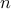# Extensions of Gauss Quadrature via Linear Programming

E. K. Ryu and S. Boyd

Foundations of Computational Mathematics, 15(4):953–971, 2015.

Gauss quadrature is a well known method for estimating the integral of a continuous function with respect to a given measure as a weighted sum of the function evaluated at a set of node points. Gauss quadrature is traditionally developed using orthogonal polynomials. We show that Gauss quadrature can also be obtained as the solution to an infinite dimensional linear program: Minimize theth moment, among all nonnegative measures that match the 0 through n-1 moments of the given measure. While this infinite dimensional linear program provides no computational advantage in the traditional setting of integration on the real line, it can be used to construct Gauss-like quadratures in more general settings, including arbitrary domains in multiple dimensions.